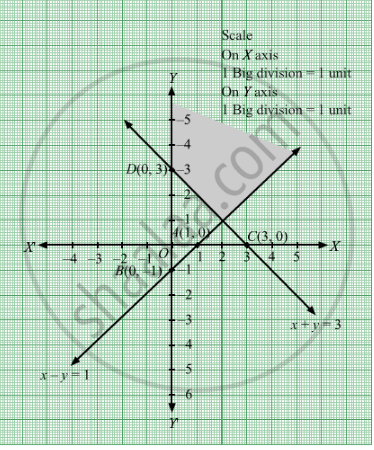# Maximize Z = 3x + 3y, If Possible, Subject to the Constraints X − Y ≤ 1 X + Y ≥ 3 X , Y ≥ 0 - Mathematics

Maximize Z = 3x + 3y, if possible,
Subject to the constraints

$x - y \leq 1$
$x + y \geq 3$
$x, y \geq 0$

#### Solution

First, we will convert the given inequations into equations, we obtain the following equations:
x − y = 1, x + y = 3, x = 0 and y = 0

Region represented by x − y ≤ 1:
The line x − y = 1 meets the coordinate axes at A(1, 0) and B(0, −1) respectively. By joining these points we obtain the line x − y = 1.
Clearly (0,0) satisfies the inequation x + y ≤ 8. So,the region in xy plane which contain the origin represents the solution set of the inequation x − y ≤ 1.

Region represented by x + y ≥ 3:
The line x + y = 3 meets the coordinate axes at C(3, 0) and D(0, 3) respectively. By joining these points we obtain the line x + y = 3.
Clearly (0,0) satisfies the inequation x + y ≥ 3. So,the region in xy plane which does not contain the origin represents the solution set of the inequation x + y ≥ 3.

Region represented by x ≥ 0 and y ≥ 0:
Since, every point in the first quadrant satisfies these inequations. So, the first quadrant is the region represented by the inequations x ≥ 0 and ≥ 0.

The feasible region determined by the system of constraints x − y ≤ 1, x + y ≥ 3, x ≥ 0 and y ≥ 0 are as follows.The feasible region is unbounded.We would obtain the maximum value at infinity.
Therefore,maximum value will be infinity i.e. the solution is unboundedm

Concept: Graphical Method of Solving Linear Programming Problems
Is there an error in this question or solution?

#### APPEARS IN

RD Sharma Class 12 Maths
Chapter 30 Linear programming
Exercise 30.2 | Q 21 | Page 33